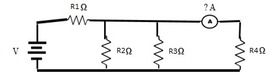# Problem 55405. Current through resistor

Three resistors (R2, R3, R4) are connected in parallel as shown in the figure. If this combination is connected in series with a battery V (volts) and a resistor R1 (ohms). Find the current I (in amps) through the R4 resistor? Round to the nearest 100th.### Solution Stats

16.93% Correct | 83.07% Incorrect
Last Solution submitted on Nov 29, 2023

### Community Treasure Hunt

Find the treasures in MATLAB Central and discover how the community can help you!

Start Hunting!